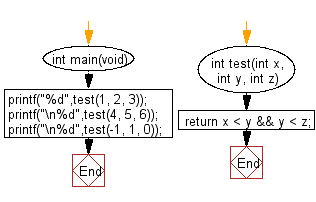﻿ C Program: Check if y>x and z>y from 3 integers x,y,z# C Exercises: Check whether y is greater than x, and z is greater than y from three given integers x,y,z

## C-programming basic algorithm: Exercise-24 with Solution

Write a C program to check whether y is greater than x, and z is greater than y from three given integers x,y,z.

C Code:

``````#include <stdio.h>
#include <stdlib.h>
int main(void){
printf("%d",test(1, 2, 3));
printf("\n%d",test(4, 5, 6));
printf("\n%d",test(-1, 1, 0));
}
int test(int x, int y, int z)
{
return x < y && y < z;
}
``````

Sample Output:

```1
1
0
```

Pictorial Presentation:Flowchart:C Programming Code Editor:

What is the difficulty level of this exercise?

Test your Programming skills with w3resource's quiz.

﻿

## C Programming: Tips of the Day

Why doesn't a+++++b work?

printf("%d",a+++++b); is interpreted as (a++)++ + b according to the Maximal Munch Rule!.

++ (postfix) doesn't evaluate to an lvalue but it requires its operand to be an lvalue.

! 6.4/4 says the next preprocessing token is the longest sequence of characters that could constitute a preprocessing token"

Ref : https://bit.ly/3fdldUT